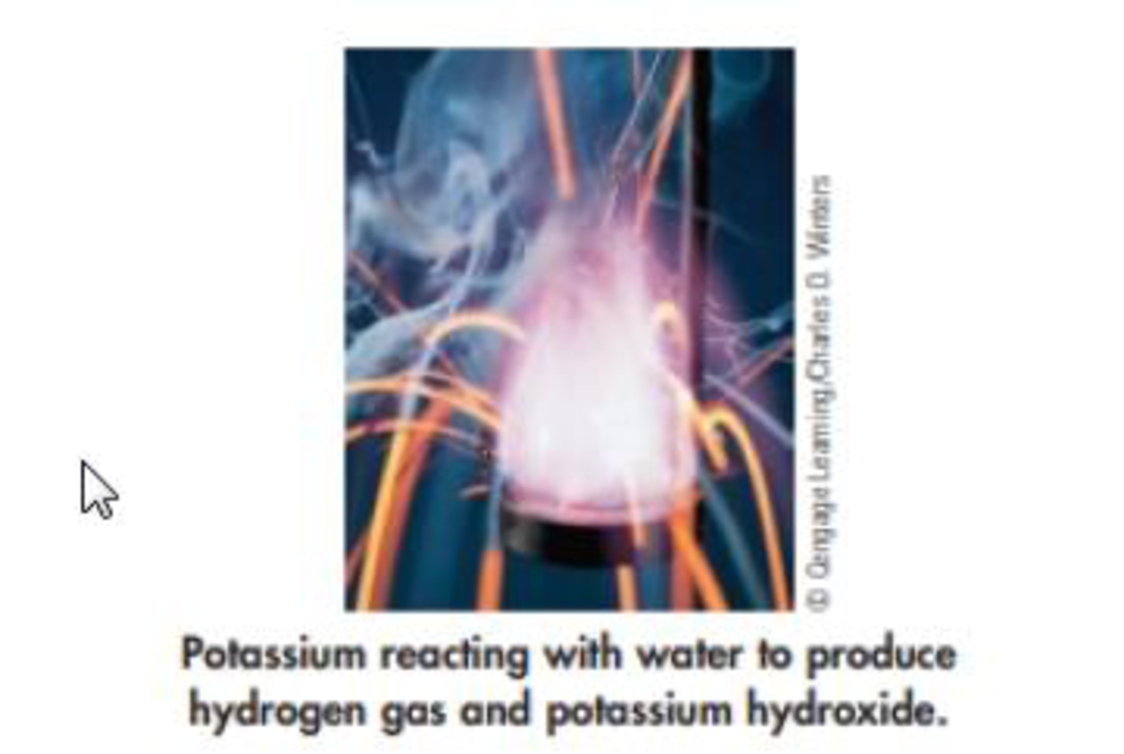Chapter 1, Problem 51GQ

Chapter
Section
Textbook Problem

The following photo shows the element potassium reacting with water to from the element hydrogen, a gas, and a solution of the compound potassium hydroxide.(a) What states of matter are involved in the reaction? (b) Is the observed change chemical or physical? (c) What are the reactants in this reaction, and what are the produces? (d) What qualitative observations can be made concerning this reaction?

(a)

Interpretation Introduction

Interpretation:

The states of matter involved in the reaction has to be given.

Explanation

States of Matter: In the given reaction process the each substance involved are Potassium ...

(b)

Interpretation Introduction

Interpretation:

The observed change has to be given.

Concept introduction:

Physical change: A change in which the substance retains in form or state without altering in composition.  It is temporary and easily reversible.

Chemical change: A change in which the substance loses its identity and produces one or more new substances by change in its composition.  It is permanent and not easily reversible.

(c)

Interpretation Introduction

Interpretation:

The reactants and the products has to be given.

(d)

Interpretation Introduction

Interpretation:

The qualitative observations made in the reactions has to be given.

Concept introduction:

Qualitative: The quality of something rather than its quantity.  A property that can be observed but not measured numerically.

Quantitative: A property that can be measured numerically and to determine the amount or proportions of its chemical constituents.

Still sussing out bartleby?

Check out a sample textbook solution.

See a sample solution

The Solution to Your Study Problems

Bartleby provides explanations to thousands of textbook problems written by our experts, many with advanced degrees!

Get Started